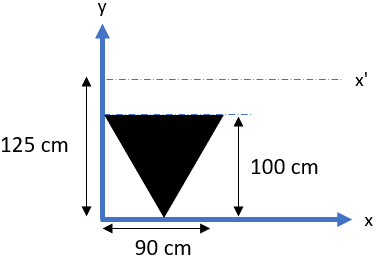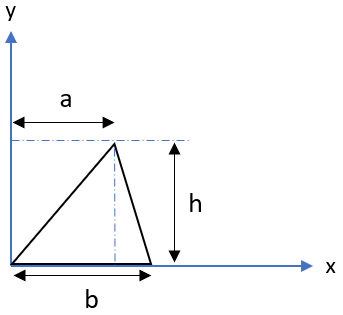## Axis Theorem

In the figure below, what is the moment of inertia about the x’ axis in m^4?Hint
Parallel Axis Theorem:
$$I_x=I_{x_{c}}+d_{y}^{2}A$$$where $$d_y$$ is the distance between the new axis and the object’s centroid, $$I_{x_{c}}$$ is the moment of inertia about the centroid axis, $$A$$ is the total cross sectional area, and $$I_x$$ is the moment of inertia about the new axis. Hint 2 The moment of inertia about the centroid axis of a triangle: $$I_{x_{c}}=\frac{bh^3}{36}$$$
where $$b$$ and $$h$$ are defined as:Parallel Axis Theorem:
$$I_x=I_{x_{c}}+d_{y}^{2}A$$$where $$d_y$$ is the distance between the new axis and the object’s centroid, $$I_{x_{c}}$$ is the moment of inertia about the centroid axis, $$A$$ is the total cross sectional area, and $$I_x$$ is the moment of inertia about the new axis. Most online tables (including the FE handbook) for area moment of inertia and centroids will have a figure similar to this:where the centroid and moment of inertia about the centroid axis are respectively defined as: $$y_c=\frac{h}{3}$$$
$$I_{x_{c}}=\frac{bh^3}{36}$$$Keep in mind that the problem is not identical to the reference figure (it’s flipped vertical). Therefore, the triangle’s centroid relative to the x-axis is actually: $$y_c=\frac{2h}{3}=\frac{2(1m)}{3}=\frac{2m}{3}=0.667\:m$$$
Since $$d_y$$ is the distance between the new axis and the object’s centroid:
$$d_y=x'-y_c=1.25m-0.667m=0.5833\:m$$$Fortunately, the moment of inertia about the centroid axis equation remains the same: $$I_{x_{c}}=\frac{(0.9m)(1m)^3}{36}=\frac{0.9m^4}{36}=0.025\:m^4$$$
Thus, the moment of inertia about the x’ axis is:
$$I_x=0.025m^4+(0.5833m)^2[\frac{1}{2}(0.9m)(1m)]=0.025m^4+0.34m^2(\frac{0.9m^2}{2})$$$$$=0.025m^4+0.153m^4=0.18\:m^4$$$
$$0.18\:m^4$$\$